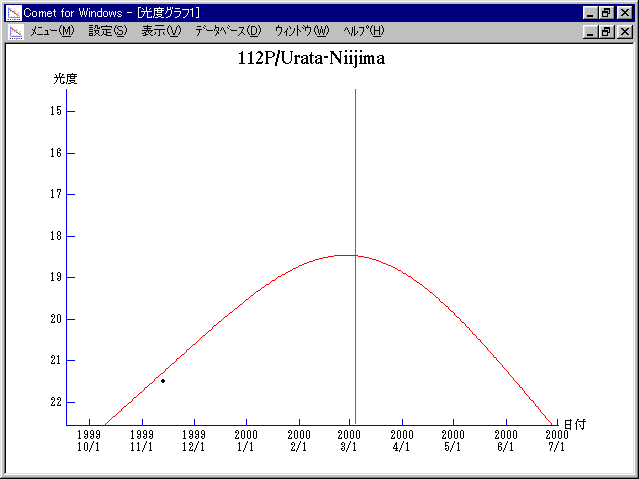# \$B1:ED(B-\$B?7EgWB@1(B

112P/Urata-Niijima (2000)###\$B50F;MWAG(B

```   The following improved orbital elements by Kenji Muraoka, are
from 87 observations  1986 to 1999, including 9 Planets, Moon
and 5 minor planets perturbations and non-gravitational effect
of style II.    The mean residual is +/- 0.87 arc seconds.

Epoch  =  2000 Feb. 26.0  TT       JDT = 2451600.5
T  =  2000 Mar.  4.38682       +/- 0.00057 (m.e.) TT
Peri. =   21.45540                +/- 0.00043
Node  =   31.94391                +/- 0.00004   (2000.0)
Incl. =   24.20477                +/- 0.00004
q  =    1.4578635              +/- 0.0000014 AU
e  =    0.5877690              +/- 0.0000003
a  =    3.5365206              +/- 0.0000013 AU
n  =    0.14819713             +/- 0.00000008
P  =    6.651                  +/- 0.0000036  years
A1  =   +0.308                  +/- 0.059
A2  =   +0.00668                +/- 0.00092
```

###\$B@1?^(B###\$B8wEYJQ2=(B

```        m1 = 12 + 5 log\$B&\$(B + 30 log r
```##### \$B50F;MWAG\$OB<2,7r<#;a\$N7W;;\$K\$h\$k\$b\$N\$G\$9!#(B \$B@1?^\$O(B StellaNavigator Ver.2.0 for Windows (\$B%"%9%H%m%"!<%D(B \$BJTCx(B / \$B%"%9%-!<=PHG6I4)(B) \$B\$G:n@.\$7\$?\$b\$N\$G\$9!#(B \$B8wEY%0%i%U\$O(BComet for Windows\$B\$G:n@.\$7\$?\$b\$N\$G\$9!#(B